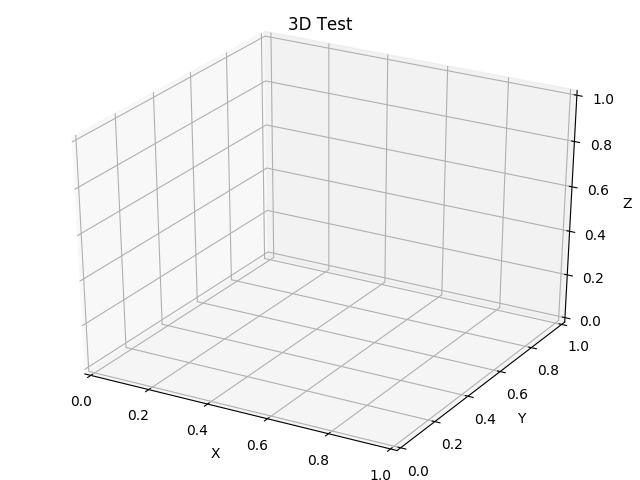Travis-CI:# 3D animation¶

An animated plot in 3D.```import numpy as np
import matplotlib.pyplot as plt
import mpl_toolkits.mplot3d.axes3d as p3
import matplotlib.animation as animation

# Fixing random state for reproducibility
np.random.seed(19680801)

def Gen_RandLine(length, dims=2):
"""
Create a line using a random walk algorithm

length is the number of points for the line.
dims is the number of dimensions the line has.
"""
lineData = np.empty((dims, length))
lineData[:, 0] = np.random.rand(dims)
for index in range(1, length):
# scaling the random numbers by 0.1 so
# movement is small compared to position.
# subtraction by 0.5 is to change the range to [-0.5, 0.5]
# to allow a line to move backwards.
step = ((np.random.rand(dims) - 0.5) * 0.1)
lineData[:, index] = lineData[:, index - 1] + step

return lineData

def update_lines(num, dataLines, lines):
for line, data in zip(lines, dataLines):
# NOTE: there is no .set_data() for 3 dim data...
line.set_data(data[0:2, :num])
line.set_3d_properties(data[2, :num])
return lines

# Attaching 3D axis to the figure
fig = plt.figure()
ax = p3.Axes3D(fig)

# Fifty lines of random 3-D lines
data = [Gen_RandLine(25, 3) for index in range(50)]

# Creating fifty line objects.
# NOTE: Can't pass empty arrays into 3d version of plot()
lines = [ax.plot(dat[0, 0:1], dat[1, 0:1], dat[2, 0:1]) for dat in data]

# Setting the axes properties
ax.set_xlim3d([0.0, 1.0])
ax.set_xlabel('X')

ax.set_ylim3d([0.0, 1.0])
ax.set_ylabel('Y')

ax.set_zlim3d([0.0, 1.0])
ax.set_zlabel('Z')

ax.set_title('3D Test')

# Creating the Animation object
line_ani = animation.FuncAnimation(fig, update_lines, 25, fargs=(data, lines),
interval=50, blit=False)

plt.show()
```

Total running time of the script: ( 0 minutes 0.077 seconds)

Gallery generated by Sphinx-Gallery